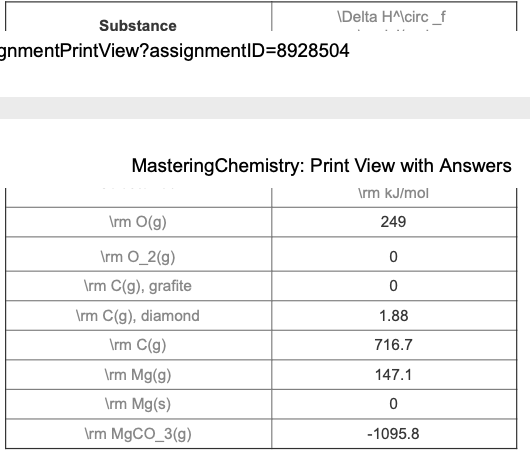# Problem: Find ΔH for MgCO3 from the given table.Express your answer using one decimal place.

###### FREE Expert Solution

Recall that ΔH˚rxn can be calculated from the enthalpy of formation (ΔH˚f) of the reactants and products involved:

$\overline{){\mathbf{∆}}{{\mathbf{H}}}_{{\mathbf{rxn}}}^{{\mathbf{°}}}{\mathbf{=}}{\mathbf{∆}}{{\mathbf{H}}}_{\mathbf{f}\mathbf{,}\mathbf{products}}^{{\mathbf{°}}}{\mathbf{-}}{\mathbf{∆}}{{\mathbf{H}}}_{\mathbf{f}\mathbf{,}\mathbf{reactants}}^{{\mathbf{°}}}}$

91% (93 ratings)###### Problem Details

Find ΔH for MgCO3 from the given table.